# Problems of the Week

Contribute a problem

Water drips into a cup (shaped like a truncated cone, as below) at a steady rate. The height of the water in the cup

• is positive, because it is above the bottom of the cup,
• has positive speed, because it is rising, and
• has negative acceleration, because the rate at which the water rises is decreasing.

How is the magnitude of acceleration of the water's height changing?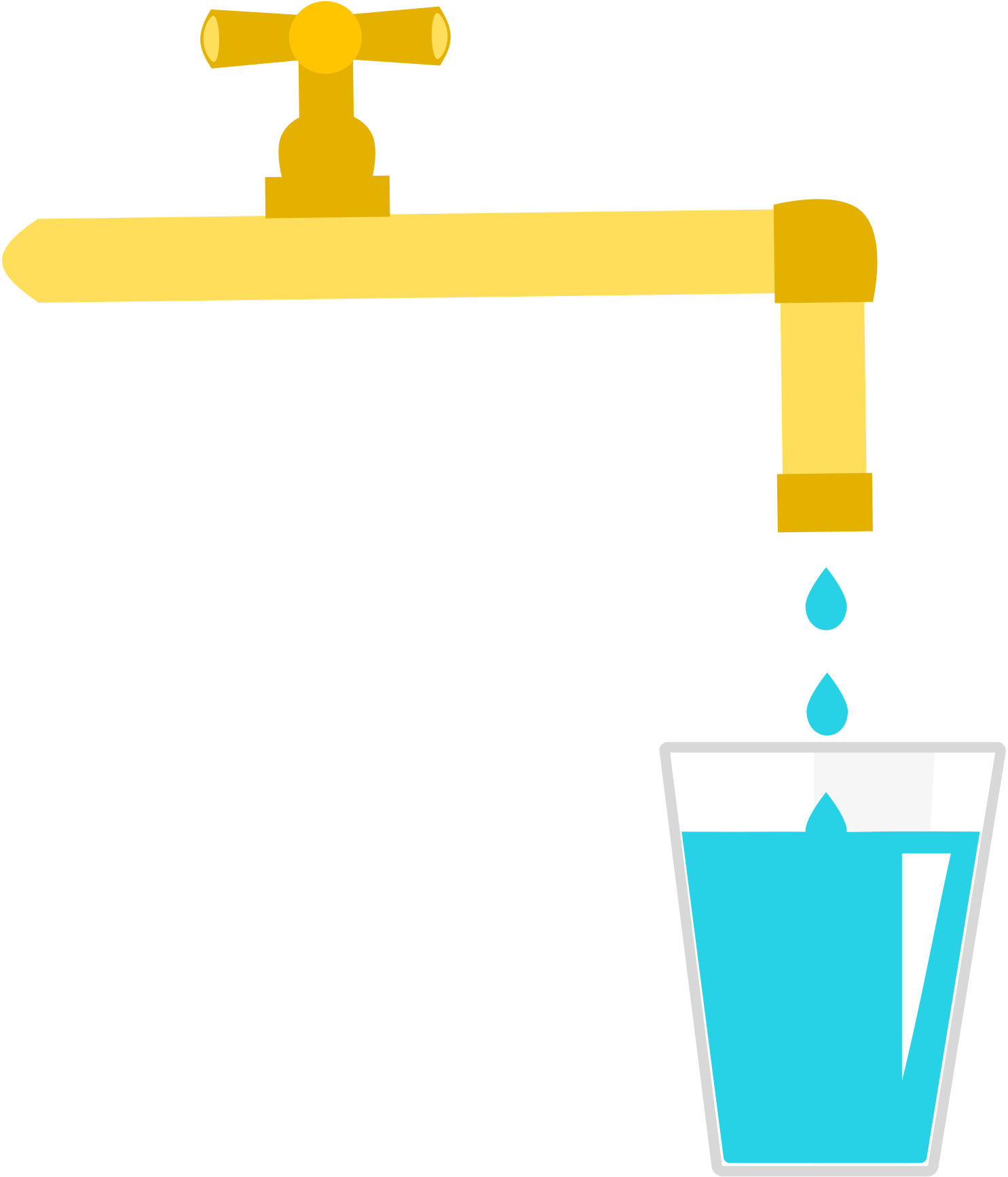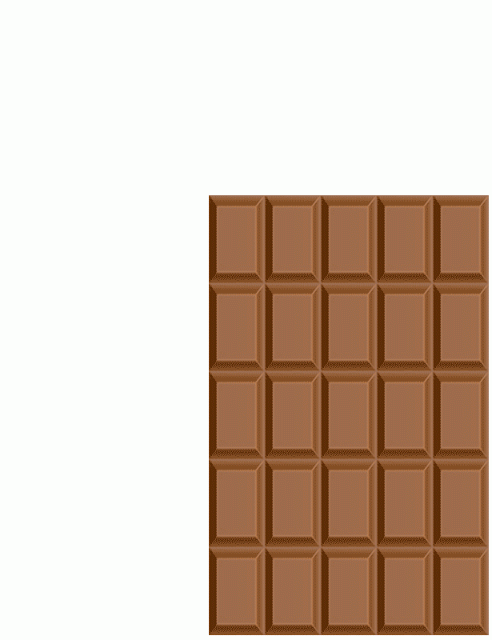The animation to the right implies that we can eat an infinite amount of chocolate from the same chocolate bar, but it is misleading—after each reassembly of the chocolate bar, the height of the chocolate bar actually decreases slightly.

Suppose you kept on cutting, taking out one whole piece while reassembling the remaining pieces into a solid rectangular bar without holes. How many whole pieces can be taken out in this way?

Let the number of whole pieces removed from the bar—out of the ten pieces labeled 1 to 10 below—be $n,$ and let the number written on the last piece removed be $x.$

What is $nx?$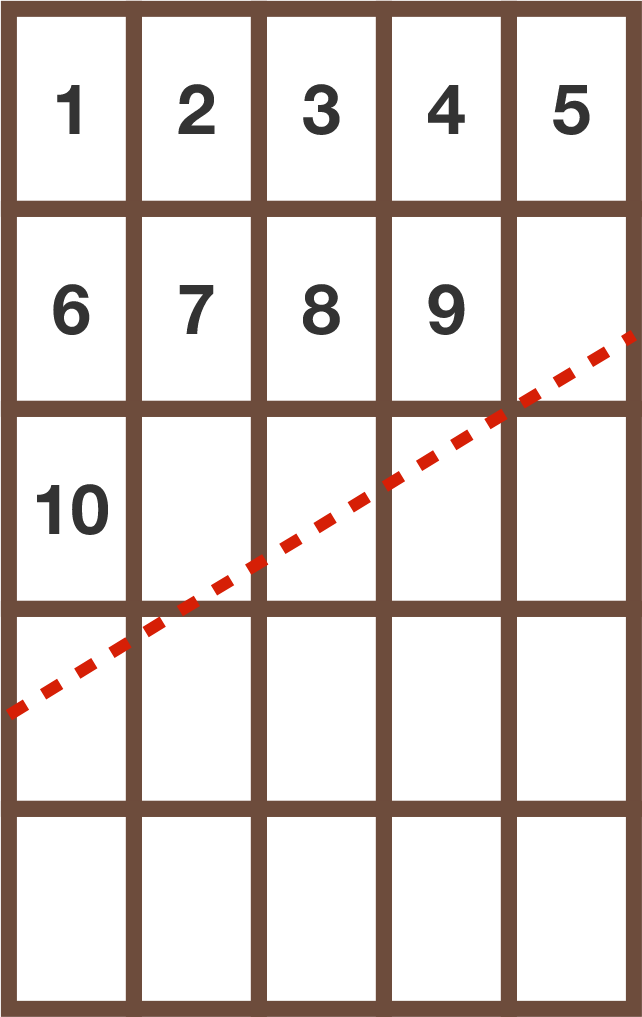Details and Assumptions:

• At the start, the sloping cut passes through the bottom right corner of piece 9, so that all the pieces below it stays the same each time the cut pieces are reassembled.
• Each reassembly is done with 3 cut pieces (out of 4), as in the animation, along and above the red, dotted line. Remember, 1 labeled piece is always eaten up after each reassembly.
• If after taking out a whole piece, the remaining pieces cannot be reassembled into a solid bar without holes, that piece does not count.

Given an ellipse (in blue) with a horizontal major axis of length 10 and a vertical minor axis of length 6, we construct a curve (in red) which is the boundary of the convex hull of points which are 3 units away from the blue ellipse.

Find the length of this red curve, and find the area of the band with width 3 (shaded in light blue) around the ellipse.

If $S$ is the sum of these two numbers, then submit $\lfloor 1000 S \rfloor$ as your answer.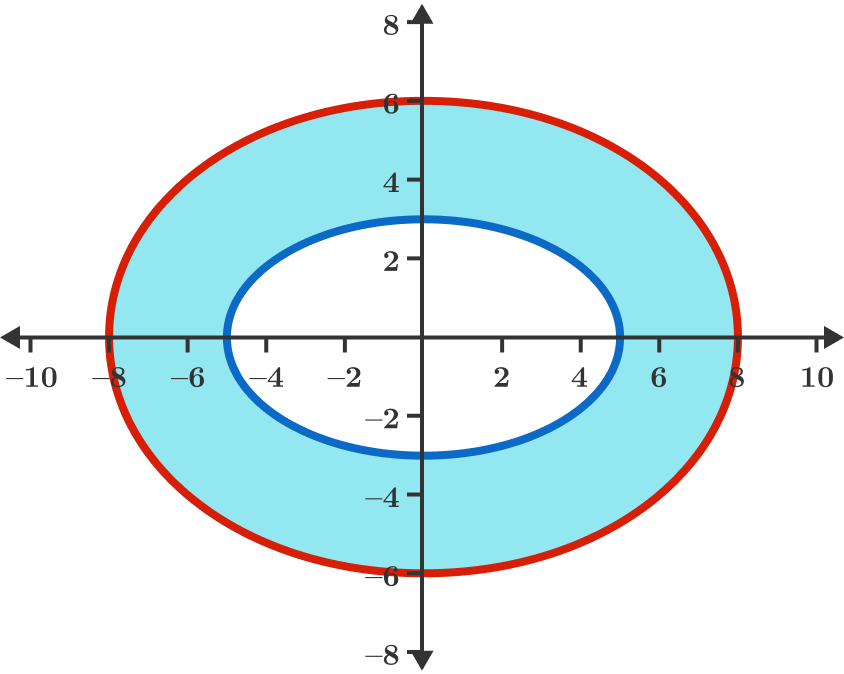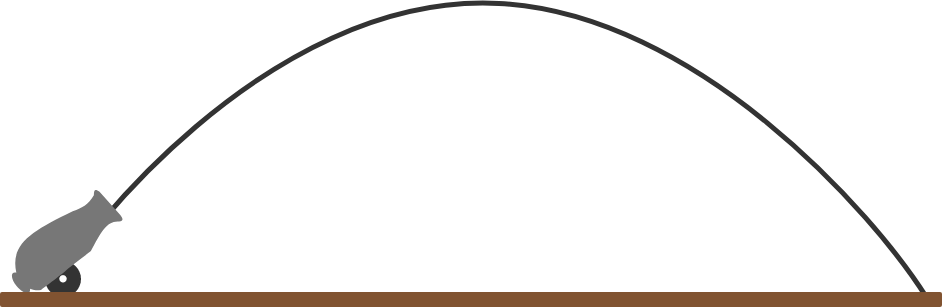A projectile is going to be fired from a cannon on level ground.

At what angle from the ground should it be fired so that it travels the maximum distance in the air?

Details and Assumptions:

• There is downward gravitational acceleration $g.$
• Neglect air resistance.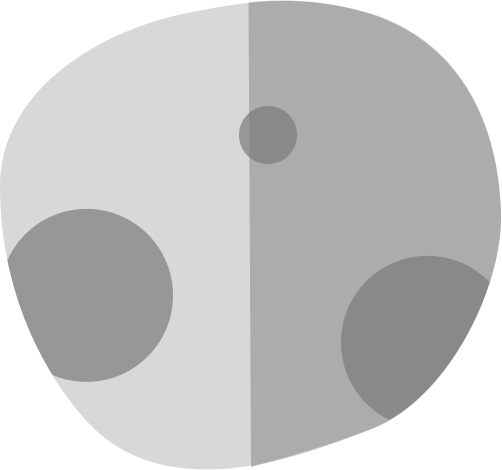You are asked to design a planet of mass $M$ and uniform density $\rho$ such that it has the maximum possible acceleration due to gravity $g$ at a point on its surface.

Find the shape of this planet, and then find $g.$

Note: $G$ is the universal gravitational constant.

×